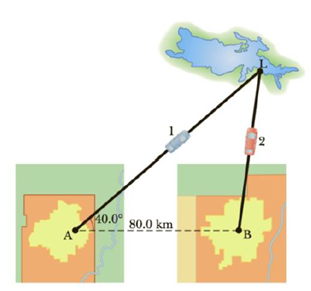Chapter 3, Problem 35AP

Chapter
Section
Textbook Problem

Towns A and B in Figure P3.35 are 80.0 km apart. A couple arranges to drive from town A and meet a couple driving from town B at the lake, L. The two couples leave simultaneously and drive for 2.50 h in the directions shown. Car 1 has a speed of 90.0 km/h. If the cars arrive simultaneously at the lake, what is the speed of car 2?Figure P3.35

To determine
The speed of car 2.

Explanation

The following diagram shows the motion of car 1 and car 2.

From the figure,

AL¯=v1t

Here,

v1 is the velocity of the first car

t is the time taken by the first car

Substitute 90.0km/h for v1 and 2.50h for t .

AL¯=(90.0km/h)(2.50h)=225km

Substitute 225km for AL¯ and 80.0km for AB¯

BD¯=(225km)cos40.0°80.0km=92

Still sussing out bartleby?

Check out a sample textbook solution.

See a sample solution

The Solution to Your Study Problems

Bartleby provides explanations to thousands of textbook problems written by our experts, many with advanced degrees!

Get Started

Find more solutions based on key concepts